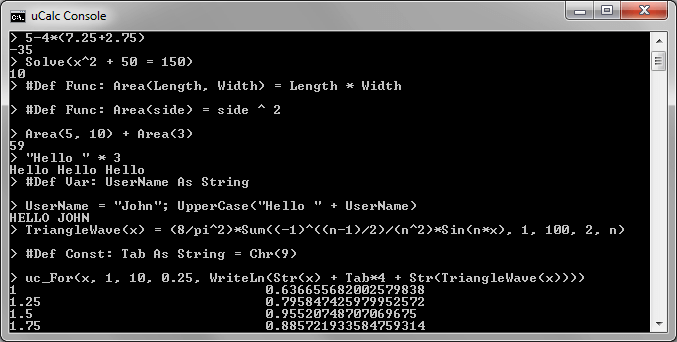#### uCalc News

graphicstores.net
Title: uCalc Console Calculator
Version: 3.00
Released: August 14, 2014
Size: 174 Kb
File: ucconsolecalc300.zip
×

### uCalc Console Calculator Demo

uCalc Console Calculator is very a powerful calculator with a simple console interface. It uses the same parser/language builder engine as other uCalc products.Here are some things you can enter at the command line:

```5-4*(7.25+2.75)

Solve(x^2 + 50 = 150)

Solve(x^2 + 50 = 150, -1000, 0)

Sum(x^2 + 25, 1, 50)

n = 25

n*2+10

g(x) = x - 1

Factorial(x) = IIf(x>1, x*Factorial(x-1), 1)

Factorial(g(12) - 5) + 10

TriangleWave(x) = (8/pi^2)*Sum((-1)^((n-1)/2)/(n^2)*Sin(n*x), 1, 100, 2, n)

#Def Const: Tab As String = Chr(9)

uc_For(x, 1, 10, 0.25, WriteLn(Str(x) + Tab*4 + Str(TriangleWave(x))))

#Def Func: Area(Length, Width) = Length * Width
#Def Func: Area(side) = side ^ 2

Area(5, 10) + Area(3)

#B11011111 OR #B10111001

#H1EFF + 25

"Hello " * 3

Rand(25, 100)

Max(1,5+4, 3+2, 89.7, 123/7, -5, 17.5)

```

For those of you who miss the Ultimate Calculator for DOS from the mid 90s, which no longer works on current Windows systems, this new calculator preserves the simple interface, but now works with Windows 10 down to Windows XP.

## Subscribe to remain up to date about uCalc

* indicates required
Join uCalc on